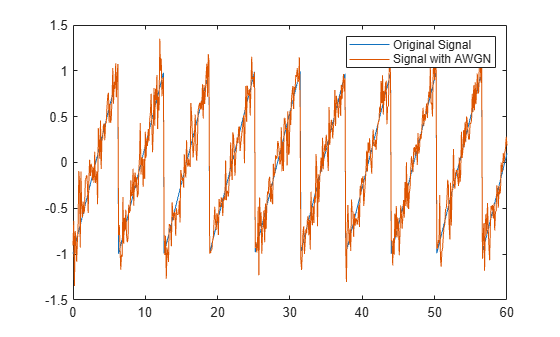awgn

Add white Gaussian noise to signal

Description

out = awgn(in,snr) adds white Gaussian noise to the vector signal in. This syntax assumes that the power of in is 0 dBW.

example

out = awgn(in,snr,signalpower) accepts an input signal power value in dBW. To have the function measure the power of in before adding noise, specify signalpower as 'measured'.

example

out = awgn(in,snr,signalpower,randobject) accepts input combinations from prior syntaxes and a random number stream object to generate normal random noise samples. For information about producing repeatable noise samples, see Tips.

out = awgn(in,snr,signalpower,seed) specifies a seed value for initializing the normal random number generator that is used when adding white Gaussian noise to the input signal. For information about producing repeatable noise samples, see Tips.

out = awgn(___,powertype) specifies the signal and noise power type as 'dB' or 'linear' in addition to the input arguments in any of the previous syntaxes.

For the relationships between SNR and other measures of the relative power of the noise, such as Es/N0, and Eb/N0, see AWGN Channel Noise Level.

Examples

collapse all

Create a sawtooth wave.

t = (0:0.1:10)';
x = sawtooth(t);

Apply white Gaussian noise and plot the results.

y = awgn(x,10,'measured');
plot(t,[x y])
legend('Original Signal','Signal with AWGN')Transmit and receive data using a nonrectangular 16-ary constellation in the presence of Gaussian noise. Show the scatter plot of the noisy constellation and estimate the symbol error rate (SER) for two different signal-to-noise ratios.

Create a 16-QAM constellation based on the V.29 standard for telephone-line modems.

c = [-5 -5i 5 5i -3 -3-3i -3i 3-3i 3 3+3i 3i -3+3i -1 -1i 1 1i];
M = length(c);

Generate random symbols.

data = randi([0 M-1],2000,1);

Modulate the data by using the genqammod function. General QAM modulation is necessary because the custom constellation is not rectangular.

modData = genqammod(data,c);

Pass the signal through an AWGN channel having a 20 dB signal-to-noise ratio (SNR).

rxSig = awgn(modData,20,'measured');

Display a scatter plot of the received signal and the reference constellation, c.

h = scatterplot(rxSig);
hold on
scatterplot(c,[],[],'r*',h)
grid
hold offDemodulate the received signal by using the genqamdemod function. Determine the number of symbol errors and the symbol error ratio.

demodData = genqamdemod(rxSig,c);
[numErrors,ser] = symerr(data,demodData)
numErrors = 1
ser = 5.0000e-04

Repeat the transmission and demodulation process with an AWGN channel having a 10 dB SNR. Determine the symbol error rate for the reduced SNR. As expected, the performance degrades when the SNR is decreased.

rxSig = awgn(modData,10,'measured');
demodData = genqamdemod(rxSig,c);
[numErrors,ser] = symerr(data,demodData)
numErrors = 462
ser = 0.2310

Generate white Gaussian noise addition results using a RandStream object and the reset object function.

Specify the power of X to be 0 dBW, add noise to produce an SNR of 10 dB, and utilize a local random stream.

S = RandStream('mt19937ar','Seed',5489);
sigin = sqrt(2)*sin(0:pi/8:6*pi);
sigout1 = awgn(sigin,10,0,S);

Add AWGN to sigin. Use isequal to compare sigout1 to sigout2. The outputs are not equal when the random stream was not reset.

sigout2 = awgn(sigin,10,0,S);
isequal(sigout1,sigout2)
ans = logical
0

Reset the random stream object, returning the object to its state prior to adding AWGN to sigout1. Add AWGN to sigin and compare sigout1 to sigout3. The outputs are equal after the random stream was reset.

reset(S);
sigout3 = awgn(sigin,10,0,S);
isequal(sigout1,sigout3)
ans = logical
1

Input Arguments

collapse all

Input signal, specified as a scalar, vector, or array. The power of the input signal is assumed to be 0 dBW.

Data Types: double
Complex Number Support: Yes

Signal-to-noise ratio in dB, specified as a scalar.

Note

When the noise is added, this function applies the same snr to all elements of the full input signal. Array input signals do not have a notion of independent channels. To consider multiple channels independently, see comm.AWGNChannel.

Data Types: double

Signal power, specified as a scalar or 'measured'.

• When signalpower is a scalar, the value is used as the signal level of in to determine the appropriate noise level based on the value of snr.

• When signalpower is 'measured', the signal level of in is computed to determine the appropriate noise level based on the value of snr.

Note

When you specify 'measured', this function computes the signal power using all elements of the full input signal. When the power is computed, array input signals do not have a notion of independent channels.

Data Types: double

Random number stream object, specified as a RandStream object. The state of the random stream object determines the sequence of numbers produced by the randn function. Configure the random stream object using the reset (RandStream) function and its properties.

wgn generates normal random noise samples using randn. The randn function uses one or more uniform values from the RandStream object to generate each normal value.

For information about producing repeatable noise samples, see Tips.

Random number generator seed value, specified as a scalar.

Data Types: double

Signal power unit, specified as 'dB' or 'linear'

• When powertype is 'dB', the snr is measured in dB and signalpower is measured in dBW.

• When powertype is 'linear', the snr is measured as a ratio and signalpower is measured in watts.

For the relationships between SNR and other measures of the relative power of the noise, such as Es/N0, and Eb/N0, see AWGN Channel Noise Level.

Output Arguments

collapse all

Output signal, returned as a scalar, vector, or array. The returned output signal is the input signal with white Gaussian noise added to it.

Tips

• To generate repeatable white Gaussian noise samples, use one of these tips:

• Provide a static seed value as an input to awgn.

• Use the reset (RandStream) function on the randobject before passing it as an input to awgn.

• Provide randobject in a known state as an input to awgn. For more information, see RandStream.

Extended Capabilities

Topics

Introduced before R2006a